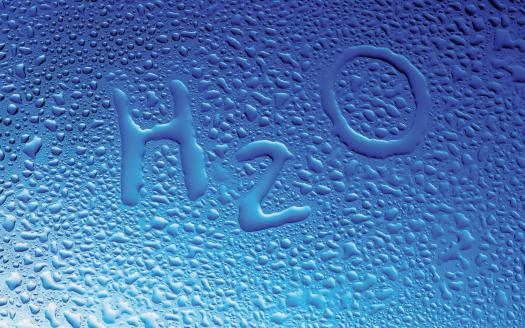# What Do You Know About Water?

Approved & Edited by ProProfs Editorial Team
The editorial team at ProProfs Quizzes consists of a select group of subject experts, trivia writers, and quiz masters who have authored over 10,000 quizzes taken by more than 100 million users. This team includes our in-house seasoned quiz moderators and subject matter experts. Our editorial experts, spread across the world, are rigorously trained using our comprehensive guidelines to ensure that you receive the highest quality quizzes.
| By Jaksiboy
J
Jaksiboy
Community Contributor
Quizzes Created: 919 | Total Attempts: 12,132,600
Questions: 10 | Attempts: 122SettingsThe earth is made up of 80% of water. You drink water when you are thirsty. Take this quiz to test how much you actually know about this compound.

• 1.

### What is the boiling point of water?

• A.

0 degree Celsius

• B.

100 degrees Celsius

• C.

250 degrees Celsius

• D.

115 degrees Celsius

B. 100 degrees Celsius
Explanation
The boiling point of water is the temperature at which it changes from a liquid to a gas. At sea level, the boiling point of water is 100 degrees Celsius. This is the temperature at which water molecules have enough energy to overcome the forces holding them together as a liquid and escape into the air as vapor.

Rate this question:

• 2.

### The solid state of water is

• A.

Hard

• B.

Ice

• C.

Rock

• D.

Water

B. Ice
Explanation
Ice is the correct answer because it is the solid state of water. When water is cooled below its freezing point of 0 degrees Celsius (32 degrees Fahrenheit), it forms ice. Ice is characterized by its hardness and solid form, making it a suitable answer for the question.

Rate this question:

• 3.

### What is the chemical formula of water?

• A.

H2O

• B.

SOH

• C.

HOH

• D.

W

A. H2O
Explanation
The chemical formula of water is H2O. This formula represents that water is composed of two hydrogen atoms bonded to one oxygen atom. The subscript 2 indicates that there are two hydrogen atoms present in the molecule, while the O represents the oxygen atom. This formula is widely recognized and used in chemistry to represent the composition of water.

Rate this question:

• 4.

### What is the freezing point of water?

• A.

100 degrees Celsius

• B.

0 degree Celsius

• C.

50 degrees Celsius

• D.

42 degrees Celsius

B. 0 degree Celsius
Explanation
Water freezes at 0 degrees Celsius. This is the temperature at which water changes from a liquid state to a solid state, forming ice. It is a well-known fact that water freezes at this specific temperature, making it the correct answer.

Rate this question:

• 5.

### Pure water is

• A.

Colourful

• B.

Colourless

• C.

Round

• D.

Slippery

B. Colourless
Explanation
Pure water is colorless because it does not contain any impurities or substances that can give it a distinct color. It appears transparent and allows light to pass through without any absorption or reflection of specific wavelengths that would create a color. Therefore, it is considered colorless.

Rate this question:

• 6.

### Water is

• A.

Oily

• B.

Opaque

• C.

Transparent

• D.

Hard

C. Transparent
Explanation
Water is transparent because it allows light to pass through it without scattering or absorbing it. This property is due to the molecular structure of water, which consists of two hydrogen atoms bonded to one oxygen atom. The arrangement of these atoms allows water to absorb only a small amount of light in the visible spectrum, making it appear clear and transparent to the human eye.

Rate this question:

• 7.

### How many oxygen atoms has the water molecule?

• A.

3

• B.

1

• C.

2

• D.

6

B. 1
Explanation
The water molecule consists of two hydrogen atoms bonded to one oxygen atom. Therefore, the correct answer is 1, as there is only one oxygen atom in a water molecule.

Rate this question:

• 8.

### Water is made up of how many elements?

• A.

1

• B.

3

• C.

2

• D.

1.5

C. 2
Explanation
Water is made up of two elements, hydrogen and oxygen. The chemical formula for water is H2O, which indicates that each water molecule consists of two hydrogen atoms bonded to one oxygen atom. Therefore, the correct answer is 2.

Rate this question:

• 9.

### The atoms that make up the water molecules are connected by

• A.

Static bond

• B.

Electricity

• C.

Covalent bond

• D.

Metals

C. Covalent bond
Explanation
Water molecules are made up of two hydrogen atoms and one oxygen atom. These atoms are connected by covalent bonds. In a covalent bond, atoms share electrons to achieve a stable electron configuration. In the case of water, the oxygen atom shares electrons with the two hydrogen atoms, resulting in a stable molecule. This sharing of electrons creates a strong bond between the atoms, allowing water to have its unique properties such as high boiling point, surface tension, and ability to dissolve many substances.

Rate this question:

• 10.

### The gaseous state of water is

• A.

Water

• B.

Steam

• C.

Flow

• D.

Air

B. Steam
Explanation
The gaseous state of water is steam. When water is heated to its boiling point, it undergoes a phase change and transforms into steam. Steam is composed of water vapor, which is water in its gaseous form. It is invisible and lighter than air, and can be used as a source of energy in various applications such as power generation and heating systems.

Rate this question:

Related TopicsBack to top